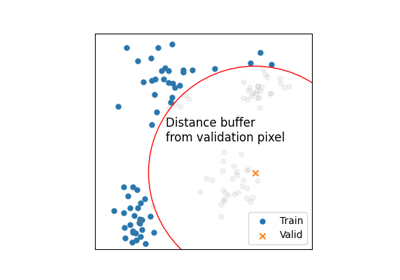# SpatialLeaveOneOut¶

class `museotoolbox.cross_validation.``SpatialLeaveOneOut`(distance_thresold=None, distance_matrix=None, n_repeats=False, n_splits=False, random_state=False, verbose=False, **kwargs)[source]

Generate a Cross-Validation with a stratified spatial Leave-One-Out method.

Parameters
• distance_matrix (numpy.ndarray, shape [n_samples, n_samples]) – Array got from function museotoolbox.vector_tools.get_distance_matrix(inRaster,inVector)

• distance_thresold (int.) – In pixels.

• n_repeats (int or False, optional (default=False)) – If False : will iterate as many times as the smallest number of groups. If int : will iterate the number of times specified.

• random_state (int or False, optional (default=False)) – If int, random_state is the seed used by the random number generator; If None, the random number generator is created with `time.time()`.

• verbose (integer or False, optional (default=False)) – Controls the verbosity: the higher the value is, the more the messages are detailed.

`museotoolbox.vector_tools.get_distance_matrix`

to get distance matrix and label.

References

See “Spatial leave‐one‐out cross‐validation for variable selection in the presence of spatial autocorrelation” : https://doi.org/10.1111/geb.12161.

Manage cross-validation methods to generate the duo valid/train samples.

Methods

 `__init__`([distance_thresold, …]) Manage cross-validation methods to generate the duo valid/train samples. `get_n_splits`([X, y, groups]) Returns the number of splitting iterations in the cross-validator. `save_to_vector`(vector, field[, group, …]) Save to vector files each fold from the cross-validation. `split`(X, y[, groups]) Split the vector/array according to y and groups.

## Examples using `museotoolbox.cross_validation.SpatialLeaveOneOut`¶